Home >  > 老男孩量化金融一（Pandas与Numpy）

## 老男孩量化金融一（Pandas与Numpy）

0

Ndarray元素类型必须相同。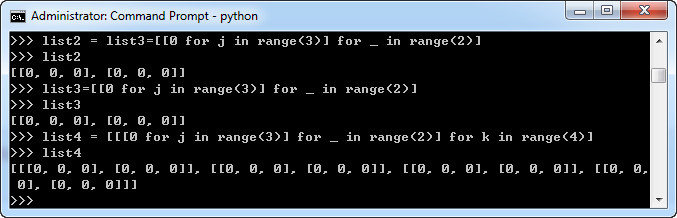```
import sys
b = np.array(range(100))
sys.getsizeof(b)

```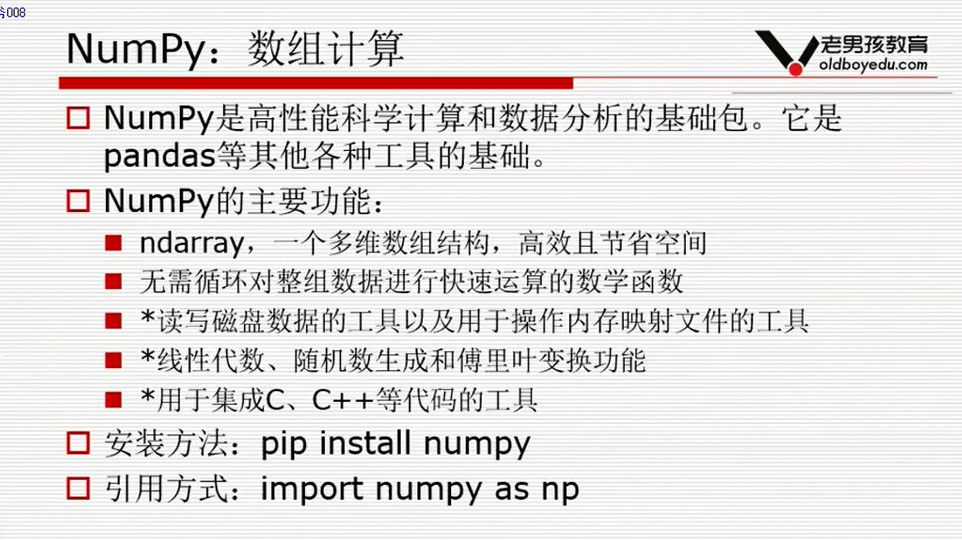```np.array([2,3,4])                 #将列表转成Ndarray
z = np.array([[1,2,3],[4,5,6]])   #多维数组
np.arange(10)                     #连续的0-9的Ndarray
np.linspace(0,10,15)              #分成15份
np.zeros(10)                      #全0的数组
np.ones(10)                       #全1的，10个1的Ndarray
np.zeros((3,5))                   #全0的3*5
np.empty()                        #随机（内存里原来的数）
a =np.arrange(15)
a =np.reshape((3,5))              #变成一个3*5的二维数组
aa[aa<5]                          #aa是一个列表 aa<5是一个布尔型数组，然后进行布尔索引

aa[[1,2,3,5,7]]                   #花式索引,取第1，2，3，5，7个元素。

a[:,[1,3]]                        #对一个二维数组，取第1列，第3列

pn.maximum(a,5)                   #取a列表，b列表每个位置最大的数

a.argmax()                        #返回最大值的下标
a.argmin()

c[~np.isnan(c)] #~表示非
```

a[2,3] 第2行第3列
ndarray元素类型必须相同。
int型数据到21亿多会越界，变成负数。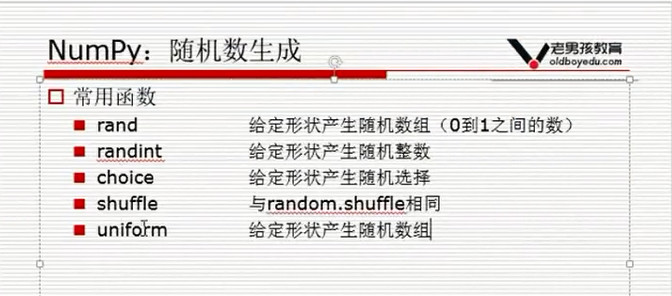np.ramdom.randint(1,10,(3,5))

1.概述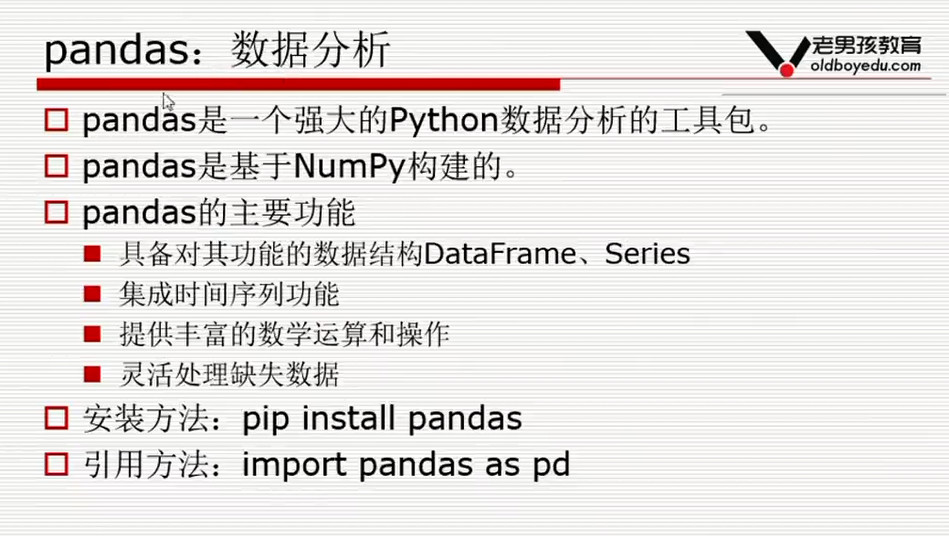2. series
series的创建方式主要有以下几种：
（1）通过一维数组方式创建
（2）从字典创建
（3）标量创建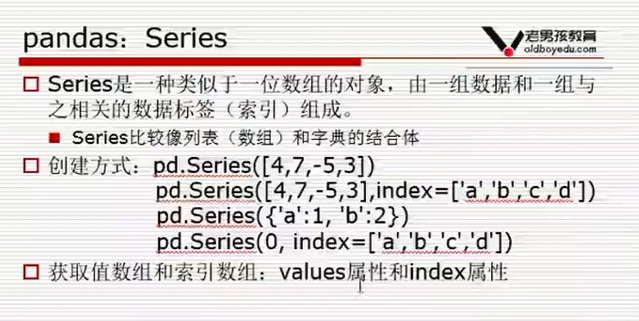（4）从ndarray创建

```data = np.array(['a','b','c','d'])
ser02 = pd.Series(data)
ser02

#指定索引
data = np.array(['a','b','c','d'])
ser02
```

```0    a
1    b
2    c
3    d
dtype: object

name       a
age        b
sex        c
dtype: object
```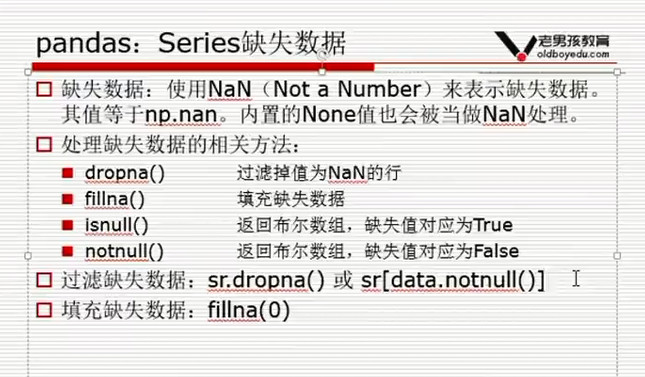3.DataFrame：
DataFrame是一个类似Excel的表格。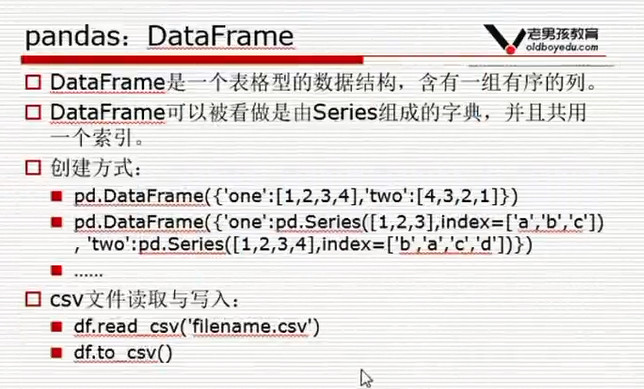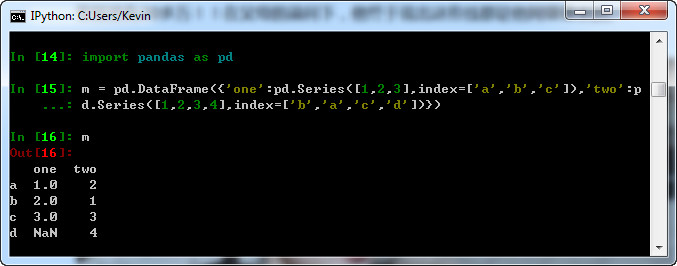```df.sort_values('close',ascending=False)  #按列排序，
df.sort_values(by='close',ascending=False)   #另一种写法
df.sort_values(['close','id'])  #按close排序，如果close相同，按id

df2.applymap(lambda x:x+1)  #将自定义函数应用在Dateframe各个元素上。

df.apply(lambda x:x.sum())  #将自定义函数应用在各行或者各列上
```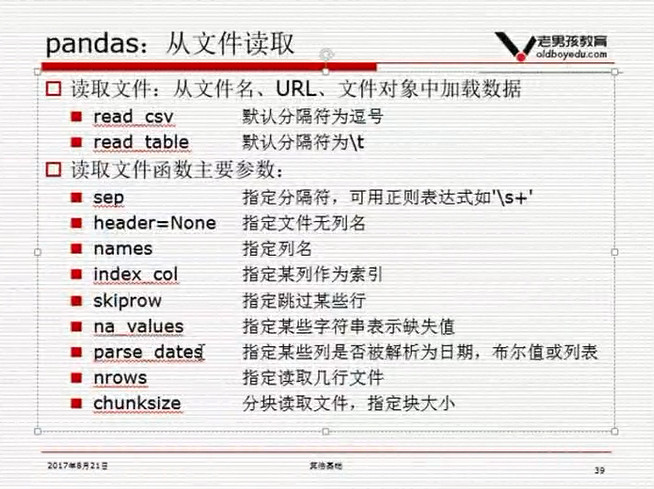```df=pd.read_table('601318.csv',sep=',',head=None,names=['id','date','open','close','high','low','volume','code'],index_col='date',parse_dates=['date'],na_values=['none','None','nan','NaN','null'])
```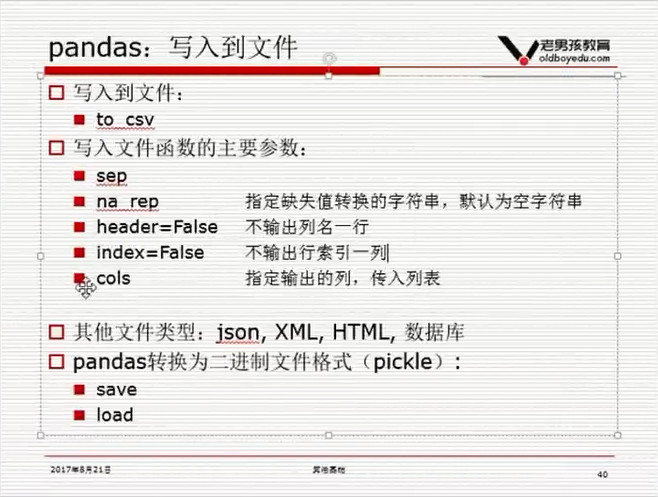```pd.date_range('2017-01-01','2017-8-1')
```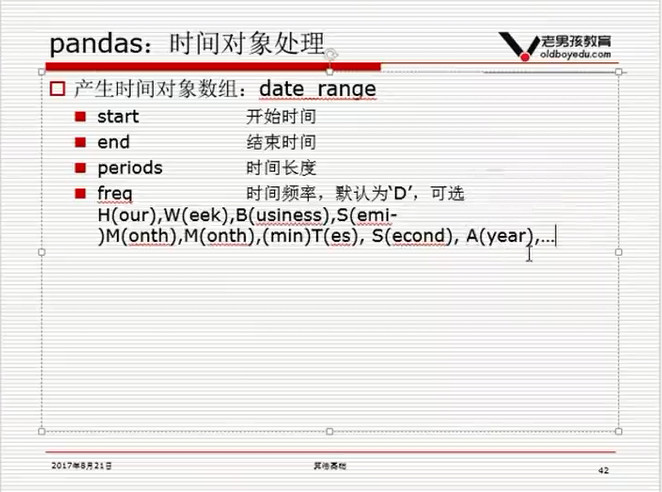4.DateFrame取数

```(1)df['close']
(2)df[df.close>10]                    #选取close>10的行
(3)df[df.index>'2017-02-13']          #大于2月13日的数据
(4)df.loc[df['close']>10,['instrument','low','high']]  #获取low大于10的各行数据 的instrument, low,high三列
(5)df.ix[:3,:2]                       #选择两列和前四行。.ix[row,colume]
(6)df.ix[:3,[x fo x in df.columns if 'width' in x]] #只选择包含width的列。
(7)df[df['class']=='Iris-virginica']  #只包含Iris-virginica类的数据

virginica = df[df['class']=='Iris-virginica'].reset_index(drop=True)
(8)df[(df['class']=='Iris-virginica') & (df['petal_width']>2.2)]   #多条件选择

(9)按行取数
b.loc   #如果索引是整数，定义按标签解释
b.iloc   #定义按下标解释  返回第10行数据（是从0开始的），df.iloc[0,0]返回第0行，第0列的数据，等同于df.ix[0,0]

df.rename(columns=('close':'new_colse'))  #给某一列改名

df[df['date'].isin(['2007-03-01','2007-03-05'])]

df.iloc[0:10,0:2]
```

pandas要求列数据的类型一样。

1.df的[]表示列，但是df[0:10]又表示行。
2.如果你要切一个小的df，可以用iloc或者 loc

loc 以标签解释。b.loc

iloc 以下标解释。b.iloc[-1]
loc，基于label的索引，loc索引的开闭区间机制和Python传统的不同，而是与MATLAB类似的双侧闭区间，即只要出现，就会包含该标签。
iloc，完全基于位置的索引。

numpy的ndarray与pandas的series和dataframe之间互转

result = ts.get_hs300s()
keywords = result['code'].tolist()

5.DateFrame计算

```df.groupby('instrument')[['amount']].sum() #按股票分组后取成交量这列数据，分别求和。
df.groupby('instrument').apply(lambda x:x.iloc)  #返回分组后的每条股票的第一条数据
df.groupby('instrument')[['amount']].sum().rename(colums={'amount':'amount_sum'}) #修改列名

df2 = pd.pivot_table(df.columns = 'instrumnet',values = 'close',index='data')   #通过pivot_table获取收盘价数据透视表
df2.shift(1)   # 索引不变，列数向后移

df["target"] = df.close.shift(-1)  #新建一个目标列，为close后移一行
df.dropna(inplace=True)

df2.rolling(3,min_periods=1).mean() #滚动窗口计算,允许最小窗口为1，因为计算第一条数据的时候没有3条
df2.expanding(().sum()   #expanding 每次从第一条数据开始算

s1 = s.fillna(0)  #s 中有nan值，用0填充
s2 = s.ffill()    #用前一天的数据填充
s3 = s.bfill()    #用后一天的数据填充

merge_df = data_df.merge(finance_df,on=['date','instrument'],how='outer')
merge_df.isnull().sum()  #查看各列缺失值行数
merge_df[merge_df.date=='2018-09-03']   #查询这一天的数据
merge_df.ffill(inplace=True)   #使用前一天数据填充
merge_df.dropna(inplace=True)  #删除前面含有Nan值的行

#通过交易日的date和instrument列，使用merge利用how='inner'，选项仅保留交易日数据
#how有inner、outer、left和right四个选项，分别表示并集，交集，按左连接和按右链接，默认为inner
data_df[['date','instrument']],merge(merge_df,on=['date','instrument'],how='inner')

```

6.表格合并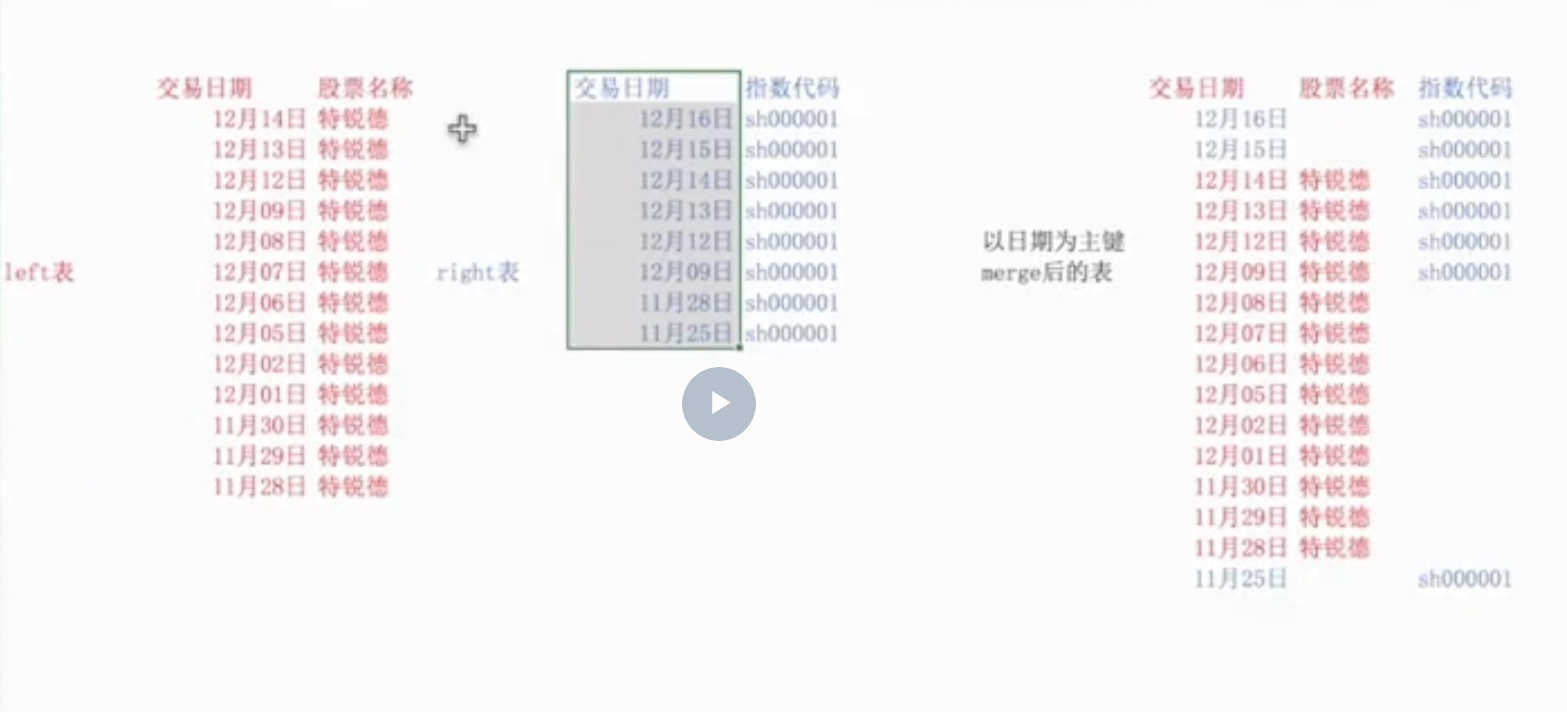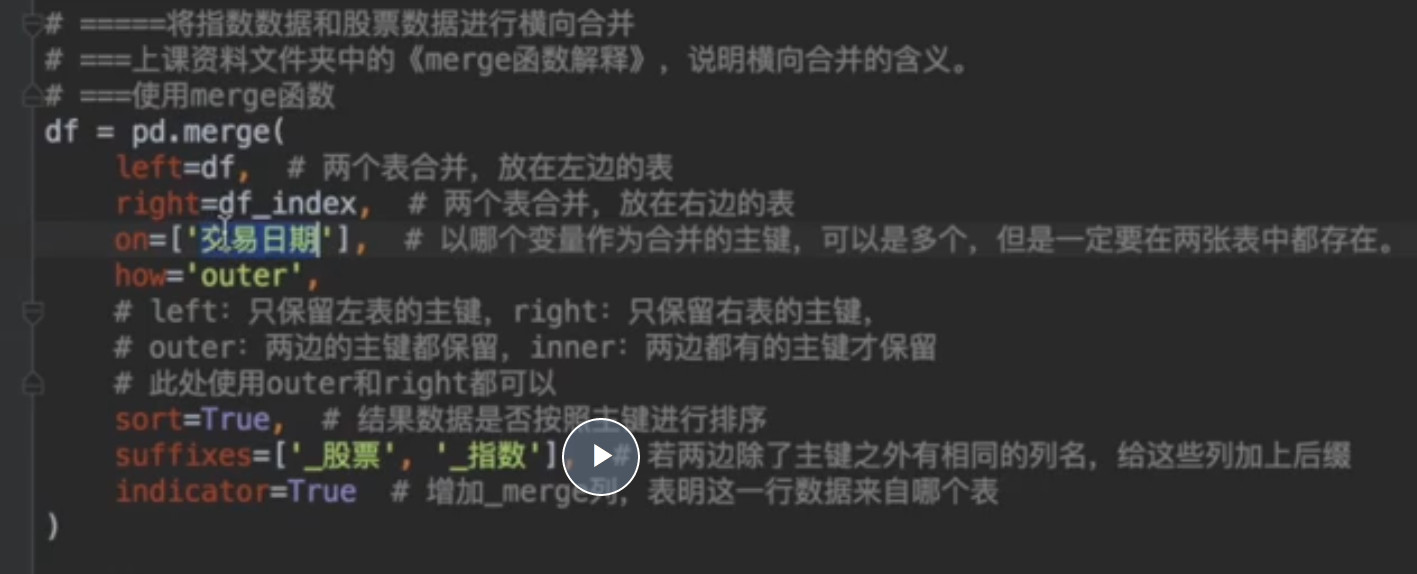7.计算天数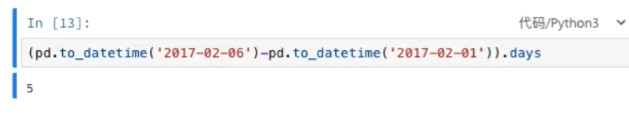```dates = pd.date_range('2/6/2017',periods=5,freq='D')
s = pd.Series([1,3,5,np.nan,6,],index= dates)

```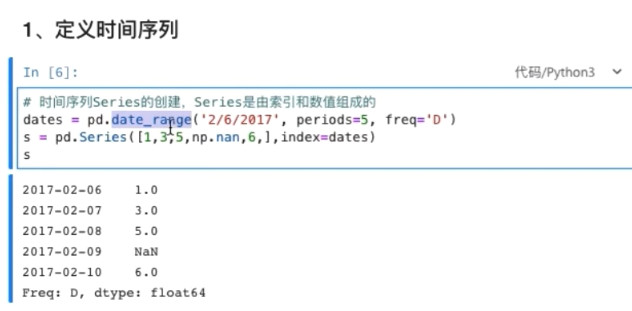8.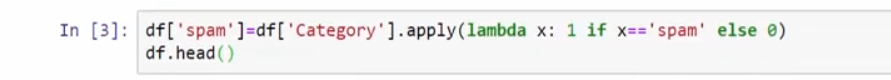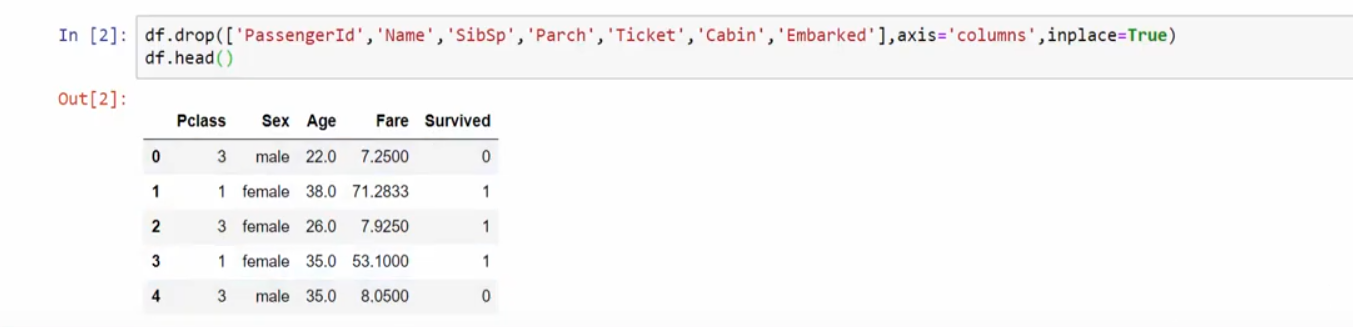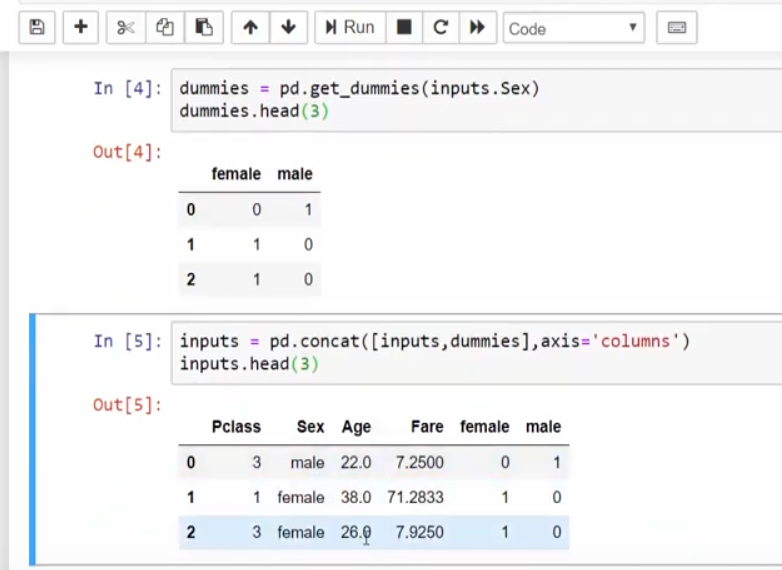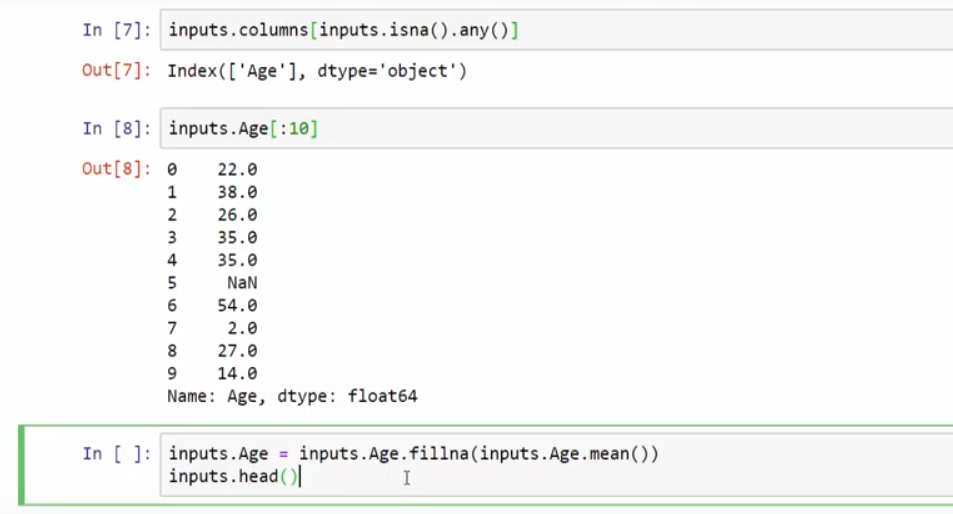print(ohlc.tail()) #显示尾5条

```#显示所有列
pd.set_option('display.max_columns', None)
#显示所有行
pd.set_option('display.max_rows', None)
#设置value的显示长度为100，默认为50
pd.set_option('max_colwidth',100)
```

```df =get_price(stock_code, start_date, end_date, frequency='30m', fields=['open','close','high', 'low'],skip_paused=False,fq='pre')
# #获取ohlc，同样带了时间的index
ohlc = df[['open', 'high', 'low', 'close']]
```

Series：一种类似于一维数组的对象，是由一组数据(各种NumPy数据类型)以及一组与之相关的数据标签(即索引)组成。仅由一组数据也可产生简单的Series对象。注意：Series中的索引值是可以重复的。

DataFrame：一个表格型的数据结构，包含有一组有序的列，每列可以是不同的值类型(数值、字符串、布尔型等)，DataFrame即有行索引也有列索引，可以被看做是由Series组成的字典。

```import pandas as pd

#更改columns名称
df.columns = [['data','open','high','low','close','volume']]
#设定为按日期索引
df.data = pd.to_datetime(df.date,fromat = '%d.%m.%Y %H:%M:%S.%f')
df = df.set_index(df.date)
```

```df.loc[condition1 & condition2, 'signal_short'] = -1  # 将产生做空信号的那根K线的signal设置为-1，-1代表做空
```

```# ===合并做多做空信号，去除重复信号
df['signal'] = df[['signal_long', 'signal_short']].sum(axis=1, skipna=True)
```

```# ===选取时间段
df = df[df['candle_begin_time'] >= pd.to_datetime('2017-01-01')]
df.reset_index(inplace=True, drop=True)
```

```# ===对每次交易进行分组
df.loc[open_pos_condition, 'start_time'] = df['candle_begin_time']
```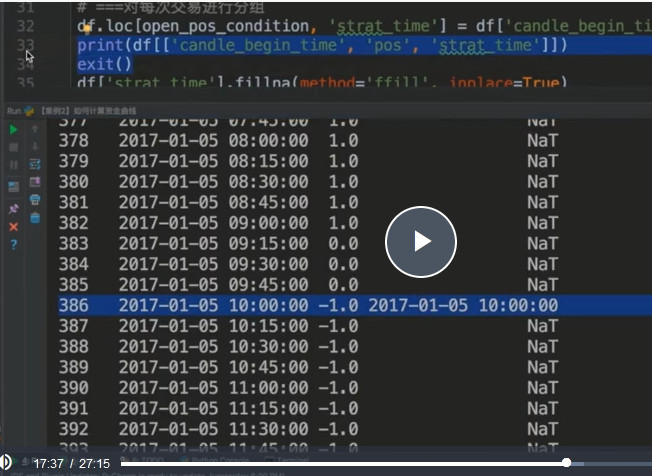```# 开仓后每天的仓位的变动
group_num = len(df.groupby('start_time'))
if group_num > 1:
t = df.groupby('start_time').apply(lambda x: x['close'] / x.iloc['close'] * x.iloc['position'])
t = t.reset_index(level=)  #将0的去掉
df['position'] = t['close']  #两个df根据index去一一赋值
```

```df = df[["close"]].copy()
```